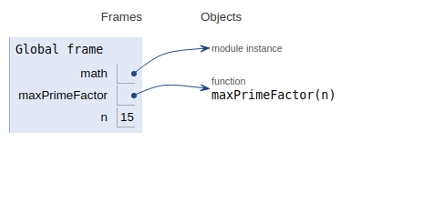# Python Program for Find largest prime factor of a number

## Problem statement

Given a positive integer n. We need to find the largest prime factor of a number.

## Approach

• Factorise the given number input by dividing it with the divisor of a number.
• Now keep updating the maximum prime factor.

## Example

Live Demo

import math
def maxPrimeFactor(n):
# number must be even
while n % 2 == 0:
max_Prime = 2
n /= 1
# number must be odd
for i in range(3, int(math.sqrt(n)) + 1, 2):
while n % i == 0:
max_Prime = i
n = n / i
# prime number greator than two
if n > 2:
max_Prime = n
return int(max_Prime)
# Driver code to test above function
n = 15
print(maxPrimeFactor(n))

Time complexity: O(n^½)

Auxiliary space: O(1)

## Output

5

All the variables are declared in the global frame as shown in the figure given below:## Conclusion